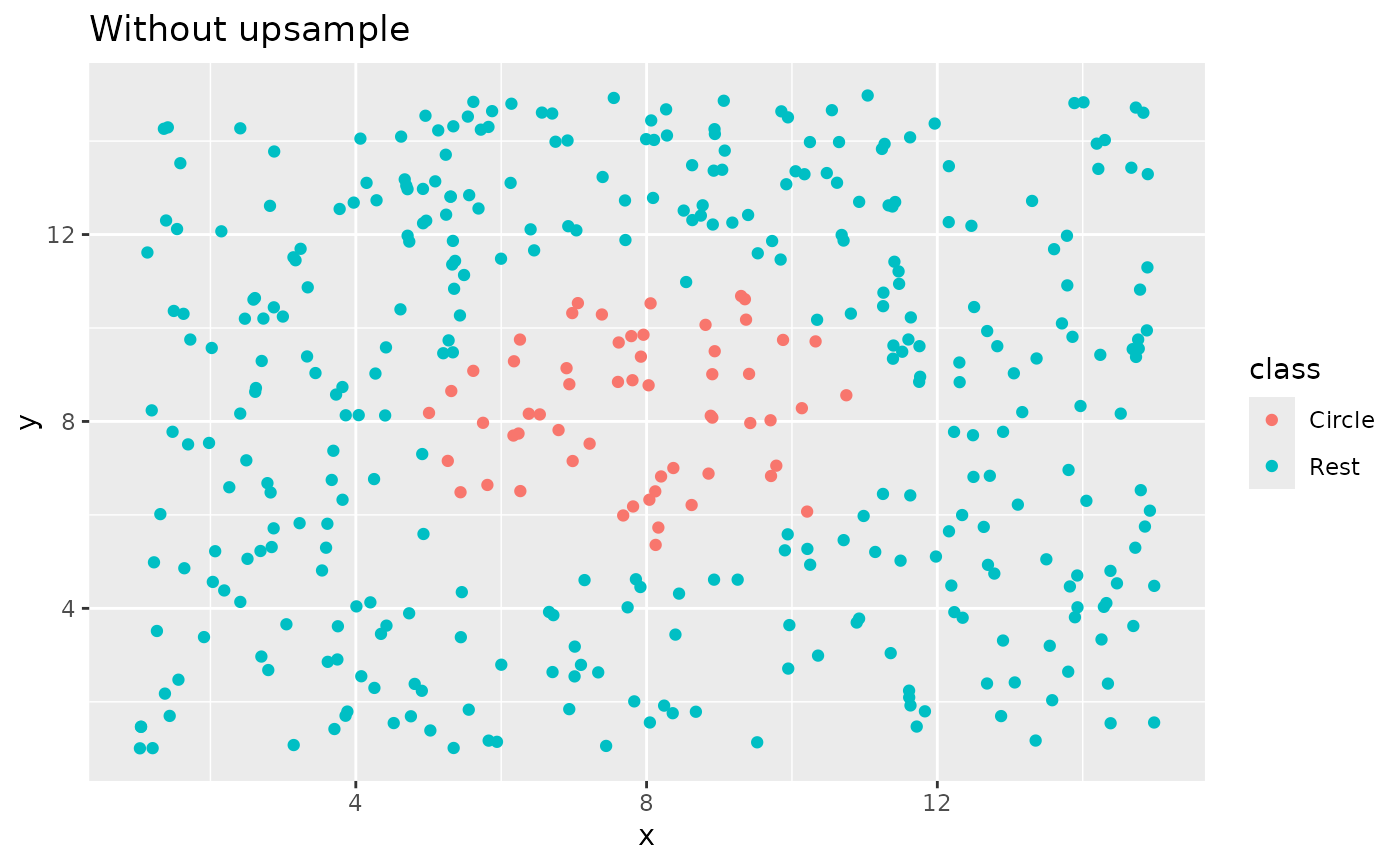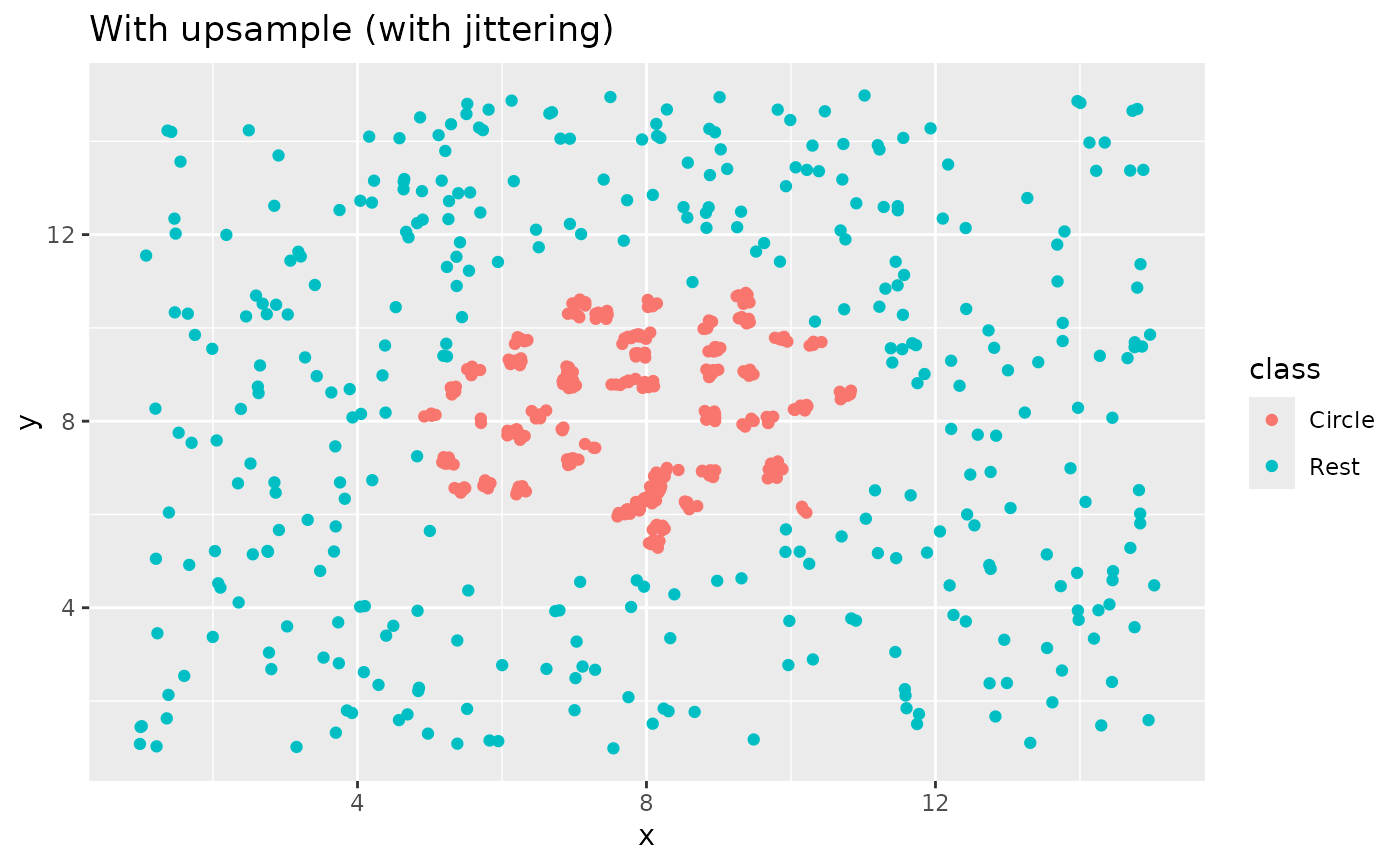step_upsample() creates a specification of a recipe step that will replicate rows of a data set to make the occurrence of levels in a specific factor level equal.

## Usage

step_upsample(
recipe,
...,
over_ratio = 1,
ratio = deprecated(),
role = NA,
trained = FALSE,
column = NULL,
target = NA,
skip = TRUE,
seed = sample.int(10^5, 1),
id = rand_id("upsample")
)

## Arguments

recipe

A recipe object. The step will be added to the sequence of operations for this recipe.

...

One or more selector functions to choose which variable is used to sample the data. See selections() for more details. The selection should result in single factor variable. For the tidy method, these are not currently used.

over_ratio

A numeric value for the ratio of the majority-to-minority frequencies. The default value (1) means that all other levels are sampled up to have the same frequency as the most occurring level. A value of 0.5 would mean that the minority levels will have (at most) (approximately) half as many rows than the majority level.

ratio

Deprecated argument; same as over_ratio.

role

Not used by this step since no new variables are created.

trained

A logical to indicate if the quantities for preprocessing have been estimated.

column

A character string of the variable name that will be populated (eventually) by the ... selectors.

target

An integer that will be used to subsample. This should not be set by the user and will be populated by prep.

skip

A logical. Should the step be skipped when the recipe is baked by bake()? While all operations are baked when prep() is run, some operations may not be able to be conducted on new data (e.g. processing the outcome variable(s)). Care should be taken when using skip = TRUE as it may affect the computations for subsequent operations.

seed

An integer that will be used as the seed when upsampling.

id

A character string that is unique to this step to identify it.

## Value

An updated version of recipe with the new step added to the sequence of existing steps (if any). For the tidy method, a tibble with columns terms which is the variable used to sample.

## Details

Up-sampling is intended to be performed on the training set alone. For this reason, the default is skip = TRUE.

If there are missing values in the factor variable that is used to define the sampling, missing data are selected at random in the same way that the other factor levels are sampled. Missing values are not used to determine the amount of data in the majority level (see example below).

For any data with factor levels occurring with the same frequency as the majority level, all data will be retained.

All columns in the data are sampled and returned by juice() and bake().

## Tidying

When you tidy() this step, a tibble with columns terms (the selectors or variables selected) will be returned.

## Tuning Parameters

This step has 1 tuning parameters:

• over_ratio: Over-Sampling Ratio (type: double, default: 1)

## Case weights

This step performs an unsupervised operation that can utilize case weights. To use them, see the documentation in recipes::case_weights and the examples on tidymodels.org.

Other Steps for over-sampling: step_adasyn(), step_bsmote(), step_rose(), step_smotenc(), step_smote()

## Examples

library(recipes)
library(modeldata)
data(hpc_data)

hpc_data0 <- hpc_data %>%
select(-protocol, -day)

orig <- count(hpc_data0, class, name = "orig")
orig
#> # A tibble: 4 × 2
#>   class  orig
#>   <fct> <int>
#> 1 VF     2211
#> 2 F      1347
#> 3 M       514
#> 4 L       259

up_rec <- recipe(class ~ ., data = hpc_data0) %>%
# Bring the minority levels up to about 1000 each
# 1000/2211 is approx 0.4523
step_upsample(class, over_ratio = 0.4523) %>%
prep()

training <- up_rec %>%
bake(new_data = NULL) %>%
count(class, name = "training")
training
#> # A tibble: 4 × 2
#>   class training
#>   <fct>    <int>
#> 1 VF        2211
#> 2 F         1347
#> 3 M         1000
#> 4 L         1000

# Since skip defaults to TRUE, baking the step has no effect
baked <- up_rec %>%
bake(new_data = hpc_data0) %>%
count(class, name = "baked")
baked
#> # A tibble: 4 × 2
#>   class baked
#>   <fct> <int>
#> 1 VF     2211
#> 2 F      1347
#> 3 M       514
#> 4 L       259

# Note that if the original data contained more rows than the
# target n (= ratio * majority_n), the data are left alone:
orig %>%
left_join(training, by = "class") %>%
left_join(baked, by = "class")
#> # A tibble: 4 × 4
#>   class  orig training baked
#>   <fct> <int>    <int> <int>
#> 1 VF     2211     2211  2211
#> 2 F      1347     1347  1347
#> 3 M       514     1000   514
#> 4 L       259     1000   259

library(ggplot2)

ggplot(circle_example, aes(x, y, color = class)) +
geom_point() +
labs(title = "Without upsample")recipe(class ~ x + y, data = circle_example) %>%
step_upsample(class) %>%
prep() %>%
bake(new_data = NULL) %>%
ggplot(aes(x, y, color = class)) +
geom_jitter(width = 0.1, height = 0.1) +
labs(title = "With upsample (with jittering)")